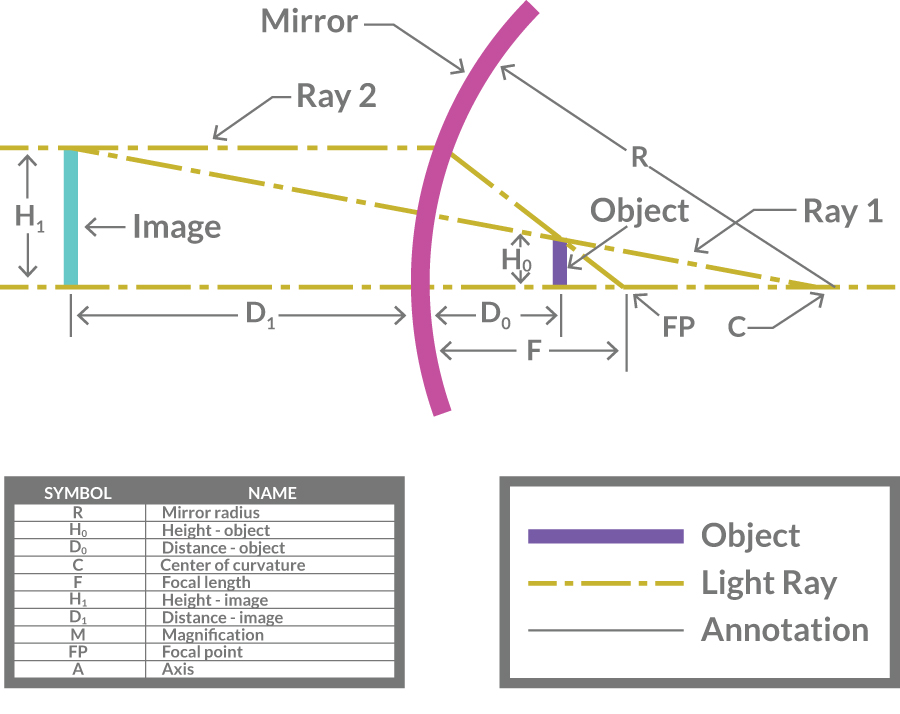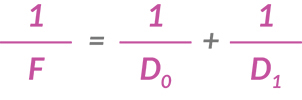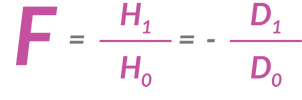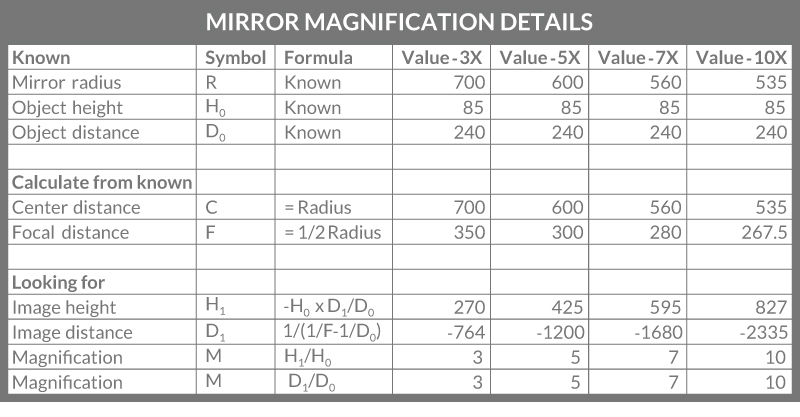### Mirror Magnification 101.

#### Let’s look at some simple points first.

1) The amount of magnification is determined by two factors;
a. The curvature of the glass – for any given mirror this is a fixed number and doesn’t change.
b. The distance of the user from the mirror, which, of course, is variable.
Skipping the details for the moment – up to a point, the further away the user gets from the mirror the larger the image.

2) Magnifying mirrors are rated for their power of magnification based on how much bigger they make an object look. A plain flat mirror would be rated at 1X and one that makes an object 3 times larger would be rated at 3X. For make‐up and shaving mirrors the most common ratings are 3X, 5X, 7X and 10X. There is a formula for the magnification but, unfortunately, it is not always applied correctly.

3) For most users a 3X or a 5X mirror works very well. Perhaps if the user’s vision is declining a bit or if they are doing very detailed work a 7X mirror would be appropriate. In a pocket size compact or other small diameter mirror a 10X magnifier works but in a larger mirror the image becomes less useful as you look further away from the center of the reflection.

4) A poorer quality mirror may show some distortion or waviness that will affect its usefulness.

5) Because its surface is harder and not as easily damaged a glass mirror will provide longer life than a plastic mirror and most metal mirrors.

6) Any mirror should be cleaned carefully. For glass mirrors use a soft, damp cloth and, if necessary a little mild detergent or glass cleaner (like Windex). Never use abrasives or scouring pads. Remember that some of the finishes on other parts of the mirror are not as damage resistant as glass and treat them accordingly. For plastic or other mirror materials refer to the manufacturer’s directions.

The simple summary is that a 3X or 5X mirror should work well for most people. When choosing a mirror, check the quality of the reflection and then decide if the magnification meets your needs. Once you have a mirror follow the manufacturer’s instructions for cleaning and maintaining it.

#### How do magnifying mirrors work – the technical stuff?

1) Mirrors function according to the laws of physics – in this case the laws of reflection.

2) Ray diagrams are a useful way to help understand how these laws work in magnifying mirrors. Basically they track the path of two light rays as they pass through specific points and are reflected from the face of the mirror. In the following diagram we are concerned with 3 points, the center of curvature, the focal point and the top of an object. Our two light rays behave as follows:
a. Ray 1 originates at the center of curvature, passes through the top of the object and is reflected back through the center of curvature – but we perceive it as passing on through the glass in a straight line.
b. Ray 2 originates at the focal point, passes through the top of the object and is reflected back parallel to the axis of curvature – but we perceive it as passing through the glass in line with this reflection.
c. The two lines intersect at a point behind the mirror which is the top of the image. The bottom of the image is a point on the axis directly below the top of the image. Because it’s on the back side of the mirror where you can’t actually see it it’s called a virtual image

3) So let’s look at a ray diagram. First let’s define some of the variables
a. R is the radius of the curved surface of the glass
b. F is the focal distance of the mirror – it is 1/2 of the radius of curvature
c. H0 is the height of the object we’re reflecting
d. D0 is the distance of the object from the center of the mirror
e. H1 is the height of its reflection
f. D1 is the distance of the reflection from the face of the mirror
g. M is the magnification of the mirror
h. R, F, H0 D0 are either known or can be fairly easily measured
i. H1 and D1 need to be calculated and once we know them there’s a simple equation for MNext let’s look at the equations for this mirror.

j. The mirror equationk. The magnification equation2) Using some high school algebra you can arrive at the data for this table (dimensions are in millimeters and numbers have been rounded)